﻿ Shock tube - XSim

# Shock tube

Update: December 17, 2018
OpenFOAM 4.x

## Case directory

\$FOAM_TUTORIALS/compressible/rhoCentralFoam/shockTube

## Summary

The shock tube is a device that generates shock wave by quick removing the diaphragm between different pressures fluids (mainly gas), which is sealed by the diaphragm.

In this tutorial, the shock tube is modeled in one dimension, with the high pressure side at 100000 Pa and the low pressure side at 10000 Pa, and the diaphragm is removed at time 0. We calculate the generating shock wave for 0.007 seconds.Model geometry

The turbulence model is not used.

The initial conditions are specified in the file system/setFieldsDict as follows.

```defaultFieldValues ( volVectorFieldValue U (0 0 0) volScalarFieldValue T 348.432 volScalarFieldValue p 100000 );
regions         ( boxToCell { box (0 -1 -1) (5 1 1) ; fieldValues ( volScalarFieldValue T 278.746 volScalarFieldValue p 10000 ) ; } );
```

And 100 sampling points on the X-axis are specified in the file constant/sample as follows. The sampling is done after the calculation.

```type sets;
libs ("libsampling.so");

interpolationScheme cellPoint;

setFormat       raw;

sets
(
line
{
type    face;
axis    x;
start   (-5 0 0);
end     (5 0 0);
nPoints 100;
}
);

fields          ( p U.component(0) T rho );
```

The mesh is shown below. The domain from -5 to 5 in X direction is divided into 100 sections.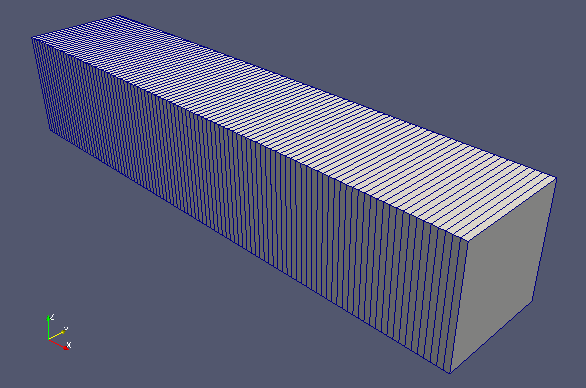Meshes

The calculation result is as follows.

The flow velocity and pressure are as follows. Note that the calculation is done in one dimension.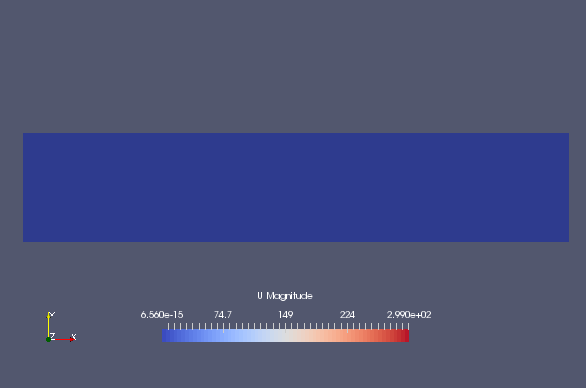Flow velocity at 0 sec (U)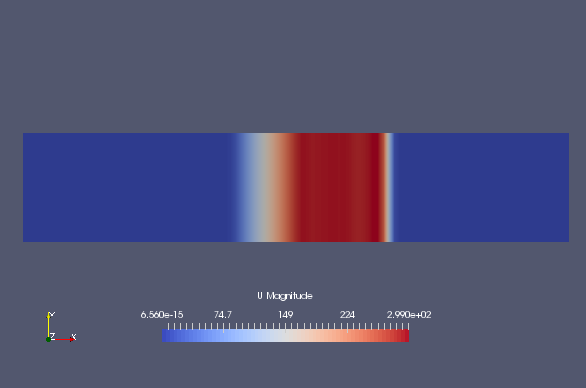Flow velocity at 0.003 sec (U)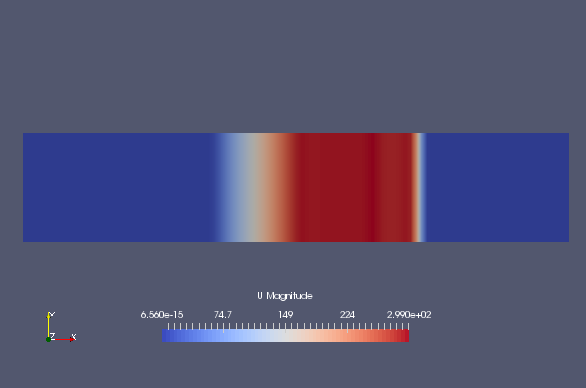Flow velocity at 0.007 sec (U)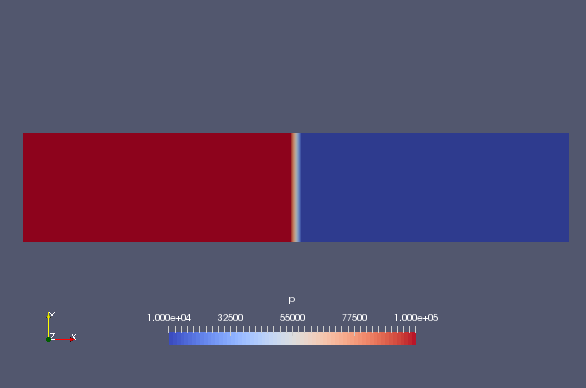Pressure at 0 sec (p)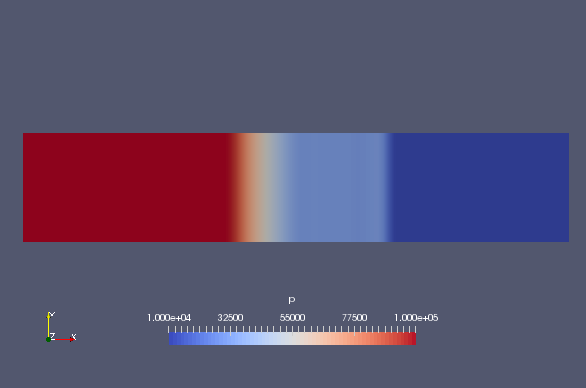Pressure at 0.003 sec (p)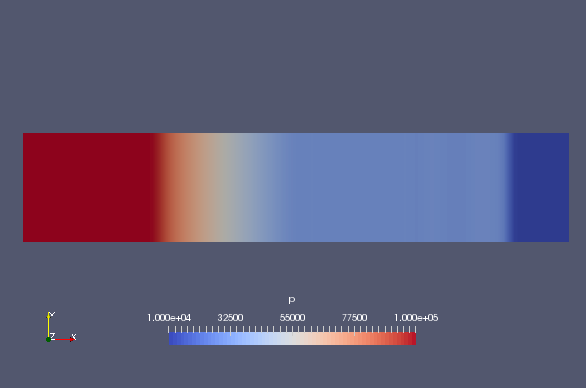Pressure at 0.007 sec (p)

The results of the sampling are as follows.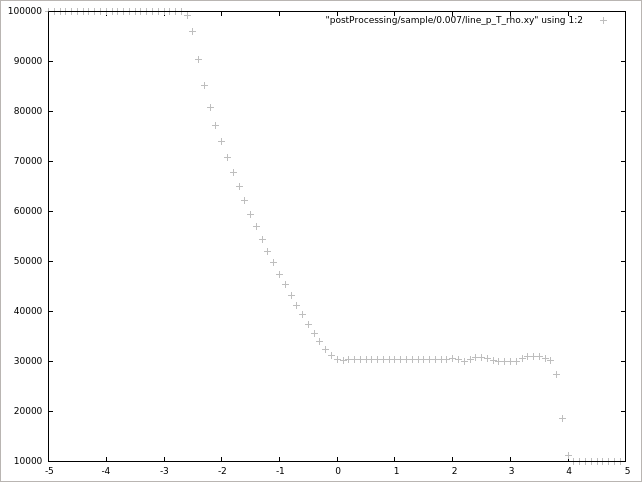Pressure at 0.007 sec (p)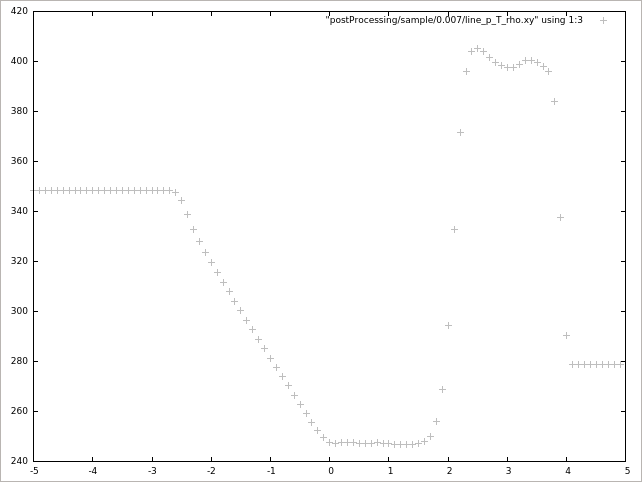Temperature at 0.007 sec (T)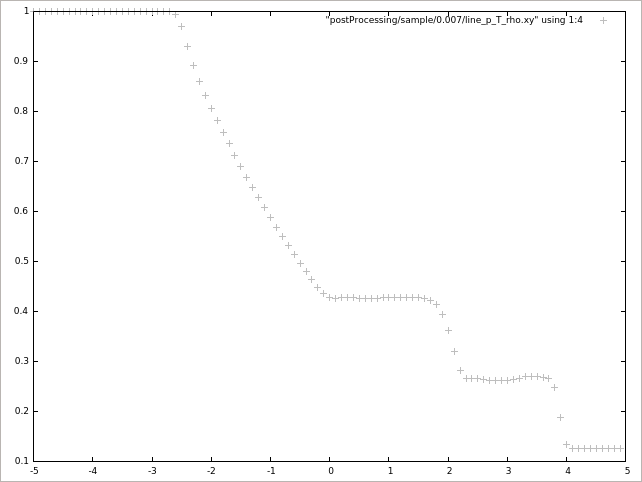Density at 0.007 sec (rho)

## Commands

cp -r \$FOAM_TUTORIALS/compressible/rhoCentralFoam/shockTube shockTube
cd shockTube

blockMesh
setFields
rhoCentralFoam
postProcess -func sample

paraFoam

gnuplot
gnuplot>plot "postProcessing/sample/0.007/line_p_T_rho.xy" using 1:2 lc rgb "gray" # pressure at 0.007 sec
gnuplot>plot "postProcessing/sample/0.007/line_p_T_rho.xy" using 1:3 lc rgb "gray" # temperature at 0.007 sec
gnuplot>plot "postProcessing/sample/0.007/line_p_T_rho.xy" using 1:4 lc rgb "gray" # density at 0.007 sec

The command "setFields" sets the initial conditions, and the command "postProcess" samples from the calculation results.

## Calculation time

0.09 seconds *Single, Inter(R) Core(TM) i7-8700 CPU @ 3.20GHz 3.19GHz